# Covariance matrix

The matrix formed from the pairwise covariances of several random variables; more precisely, for the-dimensional vector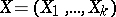the covariance matrix is the square matrix, where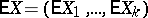is the vector of mean values. The components of the covariance matrix are: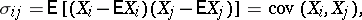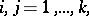and forthey are the same as(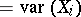) (that is, the variances of the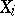lie on the principal diagonal). The covariance matrix is a symmetric positive semi-definite matrix. If the covariance matrix is positive definite, then the distribution ofis non-degenerate; otherwise it is degenerate. For the random vectorthe covariance matrix plays the same role as the variance of a random variable. If the variances of the random variables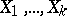are all equal to 1, then the covariance matrix ofis the same as the correlation matrix.

The sample covariance matrix for the sample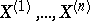, where the,, are independent and identically-distributed random-dimensional vectors, consists of the variance and covariance estimators:where the vectoris the arithmetic mean of the. If theare multivariate normally distributed with covariance matrix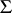, thenis the maximum-likelihood estimator of; in this case the joint distribution of the elements of the matrix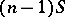is called the Wishart distribution; it is one of the fundamental distributions in multivariate statistical analysis by means of which hypotheses concerning the covariance matrixcan be tested.

How to Cite This Entry:
Covariance matrix. A.V. Prokhorov (originator), Encyclopedia of Mathematics. URL: http://www.encyclopediaofmath.org/index.php?title=Covariance_matrix&oldid=13365
This text originally appeared in Encyclopedia of Mathematics - ISBN 1402006098